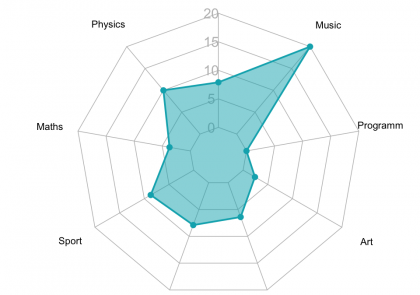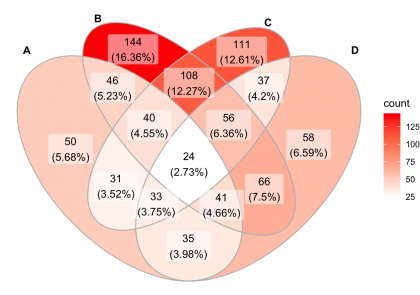# Blog

We provide practical tutorials on data mining, visualization and statistics for decision making.A radar chart, also known as a spider plot is used to visualize the values or scores assigned to an individual over multiple quantitative variables, where each variable corresponds to

#### Beautiful Radar Chart in R using FMSB and GGPlot Packages

A radar chart, also known as a spider plot is used to visualize the values or scores assigned to an individual over multiple quantitative variables, where each variable corresponds toThis article provides multiple solutions to create an elegant Venn diagram with R or RStudio. The following R packages will be illustrated: ggvenn, ggVenDiagram, VennDiagram and the gplots packages. Contents:

#### Venn Diagram with R or RStudio: A Million Ways

This article provides multiple solutions to create an elegant Venn diagram with R or RStudio. The following R packages will be illustrated: ggvenn, ggVenDiagram, VennDiagram and the gplots packages. Contents:Introduction This article describes how to create a beautiful ggplot Venn diagram. There are multiple extensions of the ggplot2 R package for creating Venn diagram in R, including the ggvenn

#### Beautiful GGPlot Venn Diagram with R

Introduction This article describes how to create a beautiful ggplot Venn diagram. There are multiple extensions of the ggplot2 R package for creating Venn diagram in R, including the ggvennThis article describes how to compute and automatically add p-values onto ggplot facets with different scales using the ggpubr and the rstatix R packages. For multipanel plots with approximately similar

#### Add P-values to GGPLOT Facets with Different Scales

This article describes how to compute and automatically add p-values onto ggplot facets with different scales using the ggpubr and the rstatix R packages. For multipanel plots with approximately similarIn this article, you will learn how to easily create a ggplot histogram with density curve in R using a secondary y-axis. We’ll use the ggpubr package to create the

#### GGPLOT Histogram with Density Curve in R using Secondary Y-axis

In this article, you will learn how to easily create a ggplot histogram with density curve in R using a secondary y-axis. We’ll use the ggpubr package to create theThis article describes how to add p-values onto horizontal ggplots using the R function stat_pvalue_manual() available in the ggpubr R package. Horizontal plots can be created using the function coord_flip()

#### How to Add P-Values onto Horizontal GGPLOTS

This article describes how to add p-values onto horizontal ggplots using the R function stat_pvalue_manual() available in the ggpubr R package. Horizontal plots can be created using the function coord_flip()#### K-Means Clustering Visualization in R: Step By Step GuideThis article describes the basics of how to compute and add p-values to basic ggplots using the rstatix and the ggpubr R packages. You will learn how to: Perform pairwise

#### How to Add P-Values onto Basic GGPLOTS

This article describes the basics of how to compute and add p-values to basic ggplots using the rstatix and the ggpubr R packages. You will learn how to: Perform pairwiseThis article describes how to compute and automatically add p-values onto ggplot facets using the ggpubr and the rstatix R packages. You will learn how to: Add p-values to a

#### How to Add P-values to GGPLOT Facets

This article describes how to compute and automatically add p-values onto ggplot facets using the ggpubr and the rstatix R packages. You will learn how to: Add p-values to aThis article describes how to display the last value of each line as a label using the ggplot2 R package. Different solutions are provided using either the ggrepel text labeling

#### GGPLOT: How to Display the Last Value of Each Line as Label

This article describes how to display the last value of each line as a label using the ggplot2 R package. Different solutions are provided using either the ggrepel text labeling